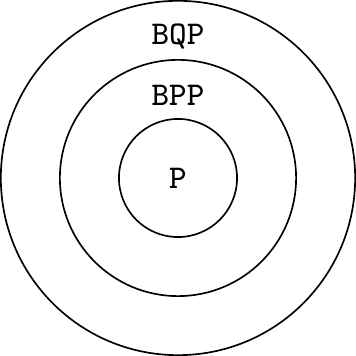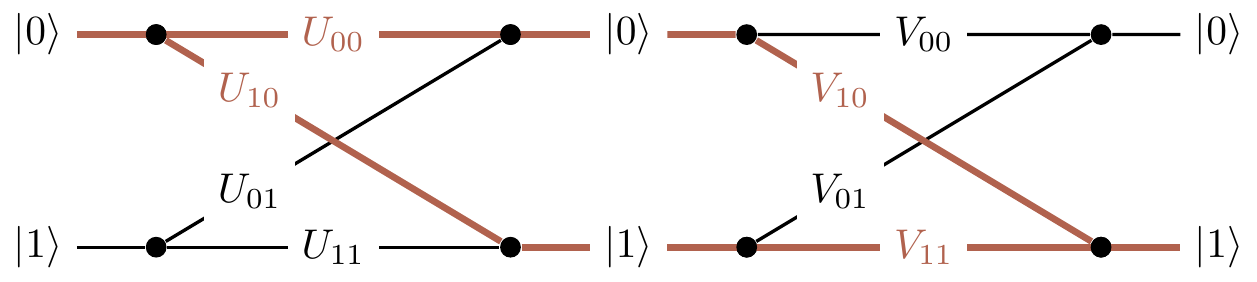## 2.1 Composing quantum operations

In order to understand something in its full complexity it is always good to start with the simplest case. Let us take a closer look at quantum interference in the simplest possible computing machine: the one that has only two distinguishable configurations — two quantum states — which we label as |0\rangle and |1\rangle. We prepare the machine in some input state, usually |0\rangle, and let it evolve: the machine undergoes a prescribed sequence of computational steps, each of which induces transitions between the two “computational states” |0\rangle and |1\rangle. The machine then ends in the output state |\psi\rangle=\alpha_0|0\rangle+\alpha_1|1\rangle, meaning the two outputs, |0\rangle and |1\rangle, are reached with probability amplitudes \alpha_0 and \alpha_1, respectively. In the process of computation each computational step U (also referred to as an operation) sends state |k\rangle to state |l\rangle, where k,l=0,1, but only with some amplitude U_{lk}. We write this as |k\rangle \longmapsto \sum_l U_{lk} |l\rangle. (watch out for the order of the indices). In words, the state |k\rangle evolves into the specific state |l\rangle with probability amplitude U_{lk} and probability |U_{lk}|^2, so the whole situation is described by the superposition (i.e. the sum) of all of these.

Thus any computational step U of this machine can be described by a matrix which tabulates all the transition amplitudes: U = \begin{bmatrix} U_{00} & U_{01} \\U_{10} & U_{11} \end{bmatrix}. The matrix element U_{lk} represents the amplitude of transition from state |k\rangle to state |l\rangle (again, watch the order of indices). To be clear, the entries in this matrix are not any random complex numbers: their moduli squared represent transition probabilities, which in turn implies that such matrices must be unitary.46

We can also describe U by drawing a diagram, which contains exactly the same information as the matrix representation, but just in a different form:Now how can we find some quantum interference to study? Consider two computational steps, U and V. What is the amplitude that input |k\rangle will generate output |m\rangle? We have to check all computational paths leading from input |k\rangle to output |m\rangle and add the corresponding amplitudes. For example, as you can see in Figure 2.1, input |0\rangle and output |1\rangle are connected by the two computational paths: |0\rangle\mapsto|0\rangle\mapsto|1\rangle (amplitude V_{10}U_{00}) and |0\rangle\mapsto|1\rangle\mapsto|1\rangle (amplitude V_{11}U_{10}). Thus the total amplitude that input |0\rangle gives output |1\rangle is the sum V_{10}U_{00}+V_{11}U_{10}, and when we take the modulus squared of this expression we will see the interference term.Figure 2.1: The composition of two computational steps, U and V, with the possible paths from |0\rangle to |1\rangle highlighted.

In general, given U and V \begin{aligned} |k\rangle &\longmapsto \sum_l U_{lk}|l\rangle \\|l\rangle &\longmapsto \sum_m V_{ml}|m\rangle \end{aligned} we can compose the two operations: we first apply U, and then V, to obtain \begin{aligned} |k\rangle &\longmapsto \sum_l U_{lk} \left( \sum_m V_{ml}|m\rangle \right) \\&= \sum_m \left( \sum_l V_{ml}U_{lk} \right) |m\rangle \\&= \sum_m (VU)_{mk} |m\rangle. \end{aligned}

If you want to hone your quantum intuition think about it the following way. The amplitude that input |k\rangle evolves to |m\rangle via a specific intermediate state |l\rangle is given by V_{ml}U_{lk} (evolutions are independent so the amplitudes are multiplied). This done, we have to sum over all possible values of l (the transition can occur in several mutually exclusive ways so the amplitudes are added) to obtain \sum_l V_{ml}U_{lk}. Thus the matrix multiplication VU (watch the order of matrices) in one swoop takes care of the multiplication and addition of amplitudes corresponding to different computational paths.

1. Recall that matrix U is called unitary if U^\dagger U = UU^\dagger = \mathbf{1} where the adjoint or Hermitian conjugate U^\dagger of any matrix U with complex entries U_{ij} is obtained by taking the complex conjugate of every element in the matrix and then interchanging rows and columns (U^\dagger_{kl}= U^\star_{lk}).↩︎